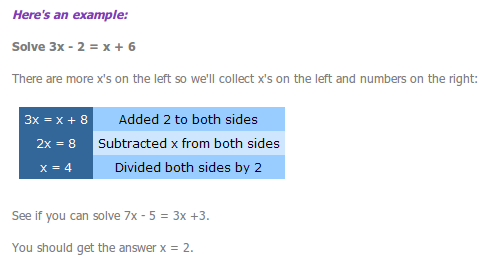# Algebra - Inequalities & Equations

• Created by: Kathryn
• Created on: 10-05-14 05:42

## Solving Linear Equations

1. Get rid of any brackets by multiplying out.
2. Get rid of any fractions by multiplying all terms by the denominator.
3. Rearrange by collecting letters on one side and numbers on the other. Remember that every term has its own sign in front of it. Negative terms need to be added to both sides to get rid of them.
4. Divide both sides by the number in front of the variable.1 of 5

ax2 +bx + c = 0

Factorising:

1. They must multiply to give 'c'.

2. They must add to give 'b'.

3. If 'c' is positive both numbers must have the same sign (both positive or both negative). If 'c' is negative then the numbers must be of opposite sign.

Quardratic Formula:If b2- 4ac is positive, there are two solutions.

If b2- 4ac is zero, there is one solution.

If b2- 4ac is negative, there are no solutions.

2 of 5

## Rearranging Formulae

Try and follow these steps:

1. Get rid of any square roots by squaring both sides.

2. Get rid of any brackets by multiplying out.

3. Get rid of any fractions by multiplying all terms by the denominator.

4. Rearrange by collecting the letter you want on one side and everything else on the other. Remember that every term has its own sign in front of it. Negative terms need to be added to both sides to get rid of them.

5. Factorise the side your variable is on so there is only one of it.

6. Divide both sides by whatever the variable is multiplied by.

3 of 5

## Simultaneous Equations

1. Rewrite both equations in the form ax + by = c

2. Match up the number of Xs or Ys in both equations by multiplying one (or both!) of the equations.

3. Merge the equations by adding them together or subtracting them. Tip! If the amount of the variable you have matched up is the same sign then subtract. If the amount of the variable is opposite signs (one positive and one negative) then add.

4. Solve the resulting linear equation.

5. Substitute your answer into one of the original equations to find the value of the other variable.

6. Put both answers into the other original equation to check they work.

4 of 5

## Inequalities

#### You can solve them in exactly the same way you solve linear equations with one extra rule that it is very important to remember!

If you multiply or divide the terms in an inequality by a negative number the inequality sign swaps round.

So, < would change to > and so on.

Simple as that! Here's an example:

Solve 12x - 5 < 8x + 3

4x - 5 < 3 (Subtracted 8x from both sides)

4x < 8 (Added 5 to both sides)

x < 2 (Divided both sides by 4 which gives us the answer!)

5 of 5